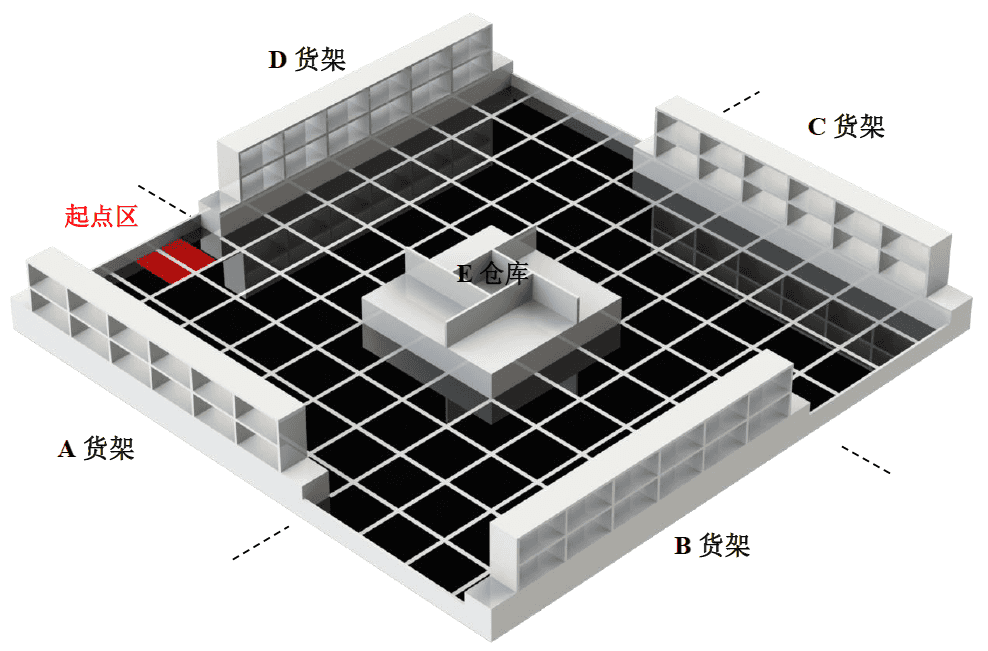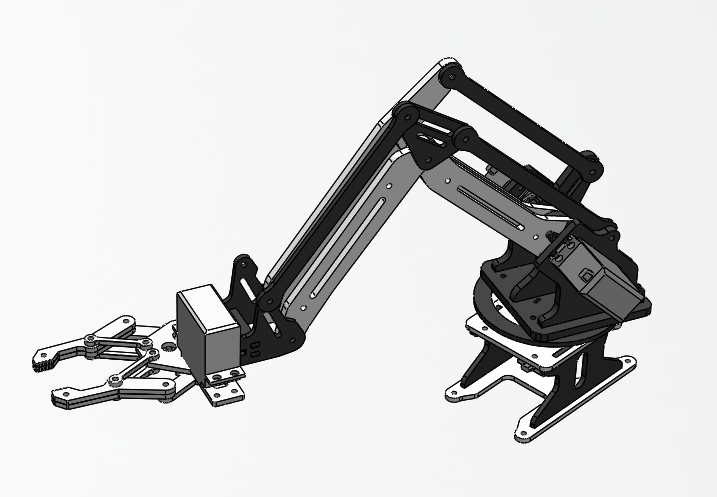# 简单四自由度机械臂的正逆向运动学求解及单片机实现

1. 1:机械臂运动学求解概述
2. 1.1:应用场景即其目的
3. 1.2:什么是运动学计算？
4. 2:机械臂结构分析
5. 2.1:约束机构分析
6. 3:正向运动学计算
7. 4:逆向运动学计算
8. 5:可行性分析
9. 6:基于单片机的C语言实现
10. 6.1:验证分析
11. 6.2:运动学求解算法的实现
12. 6.3:机械臂控制算法的实现
13. 7:效果演示
14. 8:附录
15. 8.1:附录A

1.机械臂运动学求解概述

2.机械臂结构分析

3.正向运动学计算

4.逆向运动学计算

5.可行性分析

6.基于单片机的C语言实现

7.效果演示

8.附录

## 机械臂运动学求解概述

### 应用场景即其目的### 什么是运动学计算？$$\label{一般正向运动学公式} \boldsymbol P = f \left( \theta_1 , \theta_2 , \theta_3 , \theta_4 \right)$$

$$\begin{cases} \label{一般逆向运动学公式} \theta_1 = g_1 \left( x , y, z \right) \\ \theta_2 = g_2 \left( x , y, z \right) \\ \theta_3 = g_3 \left( x , y, z \right) \\ \theta_4= g_4 \left( x , y, z \right) \end{cases}$$

$$y = f \left( x \right)$$

$$\theta_4 = f \left( \theta_1 , \theta_2 , \theta_3 \right)$$

## 机械臂结构分析### 约束机构分析$$\gamma = \frac{\pi}{2} - \alpha - \beta$$

## 正向运动学计算$$\begin{cases} d_1 = a_1 \cos b \\ d_2 = a_2 \cos s \\ d_4 = a_1 \sin b \\ d_3 = a_2 \sin s \end{cases}$$

\begin{equation} \begin{cases} \begin{aligned} x' & = d_1 + d_2 + a_3 + a_4 \\ & = a_1 \cos b + a_2 \cos s + a_3 + a_4 \\ z' & = d_4 - d_3 \\ & = a_1 \sin b - a_2 \sin s \end{aligned} \end{cases} \end{equation} \label{正向运动学方程二维}\begin{equation} \begin{cases} \begin{aligned} x & = D \cos \lambda \\ y & = D \sin \lambda \\ z & = a_1 \sin b - a_2 \sin s \\ D & = a_1 \cos b + a_2 \cos s + a_3 + a_4 \end{aligned} \end{cases} \end{equation} \label{正向运动学方程}

## 逆向运动学计算

$$\lambda = \arctan \left( \frac{y}{x} \right) \label{方位角计算公式}$$

\begin{equation} \begin{cases} \begin{aligned} x_2 & = x_4 - \left( a_3 + a_4 \right) \cos \lambda \\ y_2 & = y_4 - \left( a_3 + a_4 \right) \sin \lambda \\ z_2 & = z_4 \end{aligned} \end{cases} \end{equation} \label{P_2坐标公式}

##### 结果病态的原因

1.运算精度有限

2.除以0\begin{equation} \begin{cases} \begin{aligned}D_2 & = \sqrt{ x_2 ^2 +y_2^ 2 + z_2^2} \\ \varphi_1 & = \arccos \frac{a_1^2 + D_1^2 - a_2^2 }{2a_1D_1} \\ \varphi_3 & = \arccos \frac{a_1^2 + a_2^2 - D_1^2 }{2a_1a_1} \\ \varphi_2 & = \arctan \frac{z_2}{\sqrt{x_2^2 + y_2 ^ 2}} \end{aligned} \end{cases} \end{equation} \label{夹角求解公式}\begin{equation} \begin{cases} \begin{aligned} \angle b & = \varphi_1 + \varphi_2 \\ \angle s & = \pi - \varphi_1 - \varphi_2 - \varphi_3 \end{aligned} \end{cases} \end{equation} \label{夹角关系}